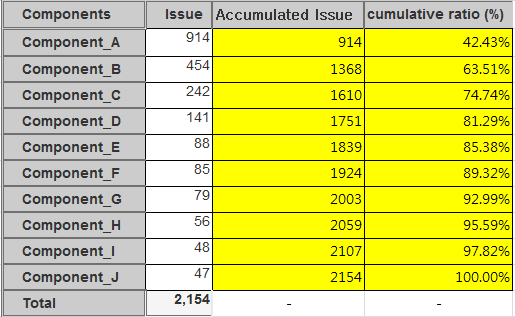# How to create the cumulative ratio

Hello, everyone.
I have a question while making a Pareto chart.

Like the yellow part of the image below,
I’d like to make a accumulative issue and a cumulative ratio.
Please give me a guide TT… (How can I create the yellow part below?)Thank you!

Such Pareto chart requires two new calculated measures: accumulated issue count over components and calculation of % from the total for each component. You may create those two measures in Measures as following.

Accumulated Issue count over components
For this calculation, use function Sum() over components which are ranked in alphabetical order. In the given example is excluded (no component) form component list as it represents issues without any component and would not provide any insight for accumulated calculation.

``````Sum(
--set of filtered components
--all components of the same project except (no component)
Filter(
Ancestor([Project].CurrentMember,[Project].[Project]).Children,
[Project].CurrentMember.Name <> '(no component)'),
--order components by name and assign rank for each
Rank(
[Project].CurrentMember,
Order(
Filter(
Ancestor([Project].CurrentMember,[Project].[Project]).Children,
[Project].CurrentMember.Name <> '(no component)'),
[Project].CurrentMember.Name,
ASC)
)),
--sum up created issues for component and previous components
[Measures].[Issues created]
)
``````

Cumulative ratio %
For this calculation, refer to the previously defined calculated measure “Accumulated Issues over Components” and divide it with total created issues for the selected project. Again, this calculation excludes (no component) from the component list to use the same set of components as in calculation “Accumulated Issues over Components.”

``````[Measures].[Accumulated Issues over Components]
/
--divide by total issues created for selected project except (no component)
Sum(
Filter(
Ancestor([Project].CurrentMember,[Project].[Project]).Children,
[Project].CurrentMember.Name <> '(no component)'),
[Measures].[Issues created])
``````

For more details on calculated measures and used function, please visit eazyBI documentation:

In the report, you may set Project dimension on rows and pages at the same time and filter components by a specific project (see picture below).

Best,
Zane / support@eazyBI.com